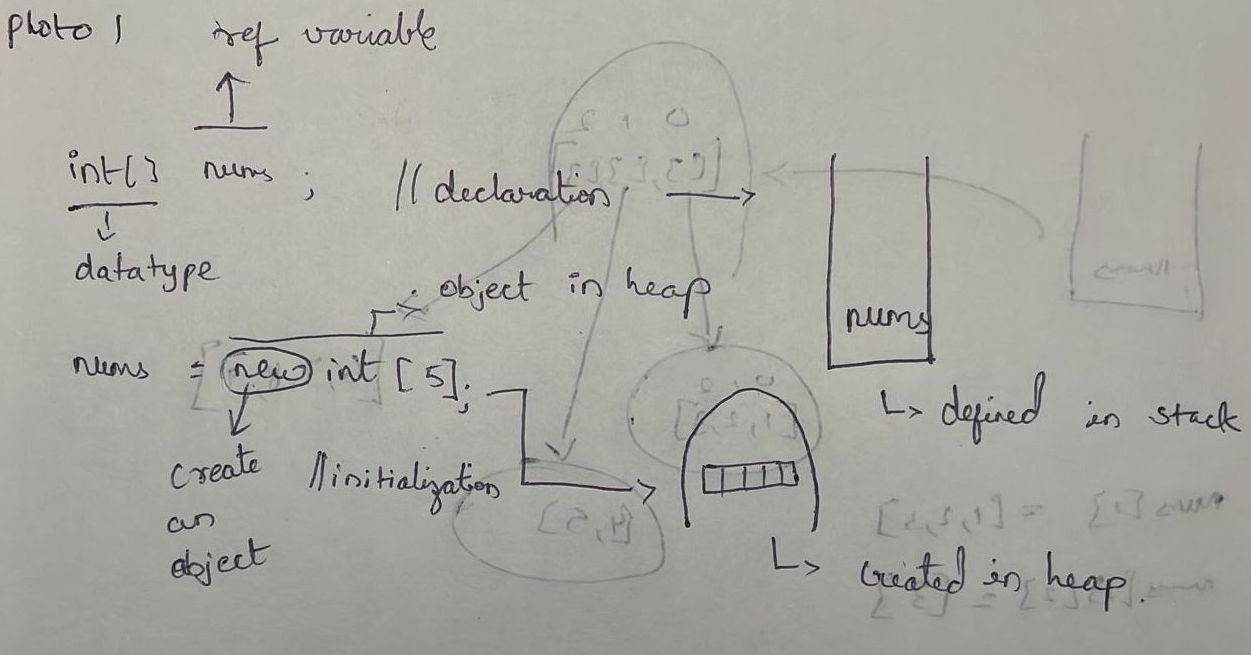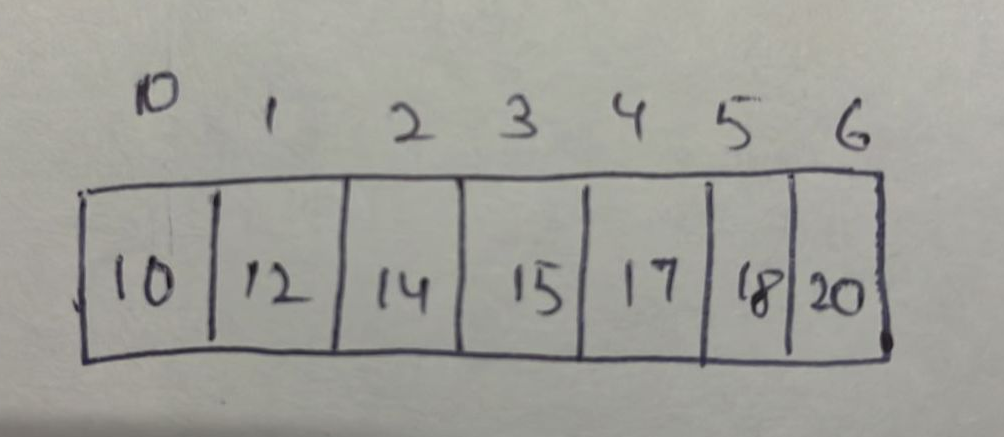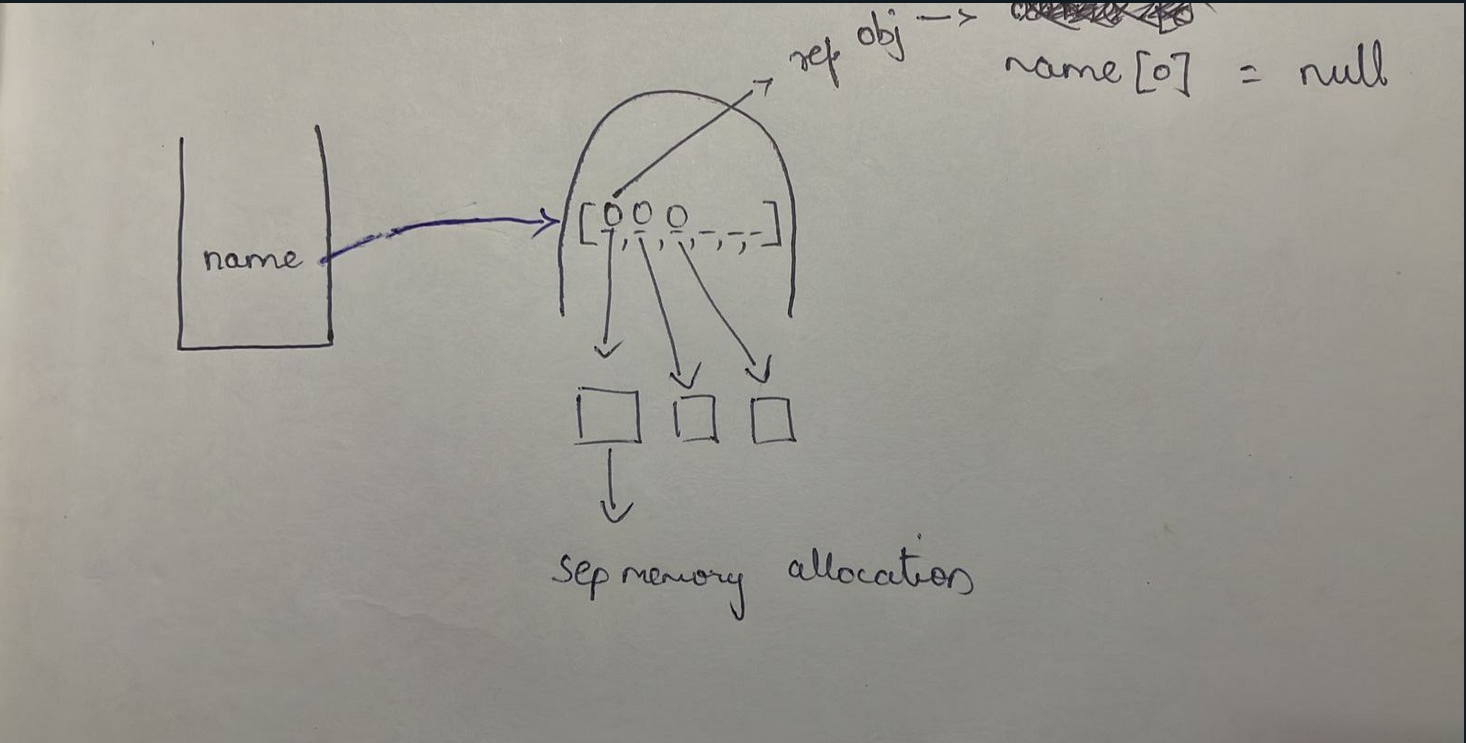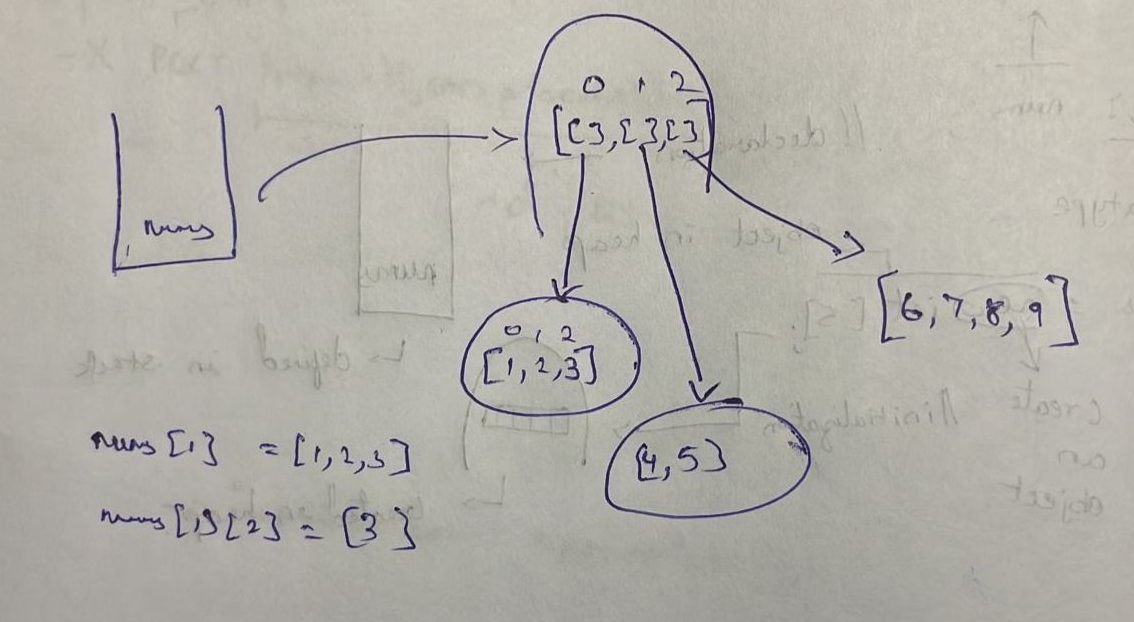# Sreekarshodhan's Blog# What is an array?

• Array is a datastructure which is a collection of datatypes, primitives basically similar elements in it.

# What is the Syntax of array?

`````` datatype[] variable_name = new datatype[size];

int[] rnos = new int
//or
int[] rnos = {1,2,3,4,5}
``````
• Note:
• int represents the datatype that is allowed to store

• All the type of data in the array must be same

• this int[] -> rnos reference variable is pointing towards an array object that contains type integer elements

• int[] rnos -> means the rnos is getting defined in the stack means declaration of array.

int[] nums; // declaration means the nums are defined in the stack

nums = new int; // initialisation means actually here object is being created in the heap

# How this works internally :

• declaration runs in compile time

• initialization or object creation runs in runtime. This is known as Dynamic memory allocation. Memory gets allocated during runtime.

•## Continuity of Array :

• Array is continous memory allocation in other languages.

• Array objects are in heap.

• Heap objects are not continous.

• Dynamic memory allocation.

• Hence array objects in java may not be continous, completely depends on JVM.

## Array index:• index are numbers which says the position of array elements. It's like roll numbers to the array elements.

int[] nums = {10,12,13,14,16,18}; sout(nums)-> prints 10 sout(nums) -> prints 18

• User: roll number 0 student10: yes sir!!! (lol I know this is funny)

User : roll number 4 student16 : yes sir!!!

(Hope you got the point)

• Initially, all the integer values for an integer array by default all the values are 0.

• for string array, by default the value is null.

• internal working of arrays:# input using for loop

``````  for(int i = 0; i < arr.length; i++){
arr[i] = in.nextInt();
}
// int i = 0 -> the input starting from 0 index of array
// i < arr.length -> condition for i is less than length of array
// i++ increament of i i.e move to next element in array
This is used if the input is very huge 1000's etc
``````

# 2D arrays in java

``````  int[][] nums = new int[size][];
// double brackets for 2D array, size for rows is compulsory but size for columns is not necessary

or

int[][] arr = {
{1,2,3},
{4,5},
{6,7,8,9},
}
// above row 1,2,3 has different no.of columns thats why size is not compulsory for columns bracket
``````# What are some important array methods?

``````   int[][] nums = new int
sout(nums.length); // ouputs no.of rows

for(int row = 0; row < arr.length; row++){
// for each colin every row
for(int col; col < arr[row].length; col){
nums[row][col] = in.nextInt();
}
}
``````
• Arrays are fixed size, what will you do when size is not known? ArrayList is the answer

• ArrayList comes under OOPS collections concept.